• matlab时频分析之短时傅里叶变换 spectrogram 短时傅里叶变换常用于缓慢时变信号的频谱分析，可以观察沿时间变化的频谱信号。 其优点如下图所示，弥补了频谱分析中不能观察时间的缺点，也弥补了时域分析不能获取频率...
matlab时频分析之短时傅里叶变换 spectrogram
短时傅里叶变换常用于缓慢时变信号的频谱分析，可以观察沿时间变化的频谱信号。
其优点如下图所示，弥补了频谱分析中不能观察时间的缺点，也弥补了时域分析不能获取频率的缺点。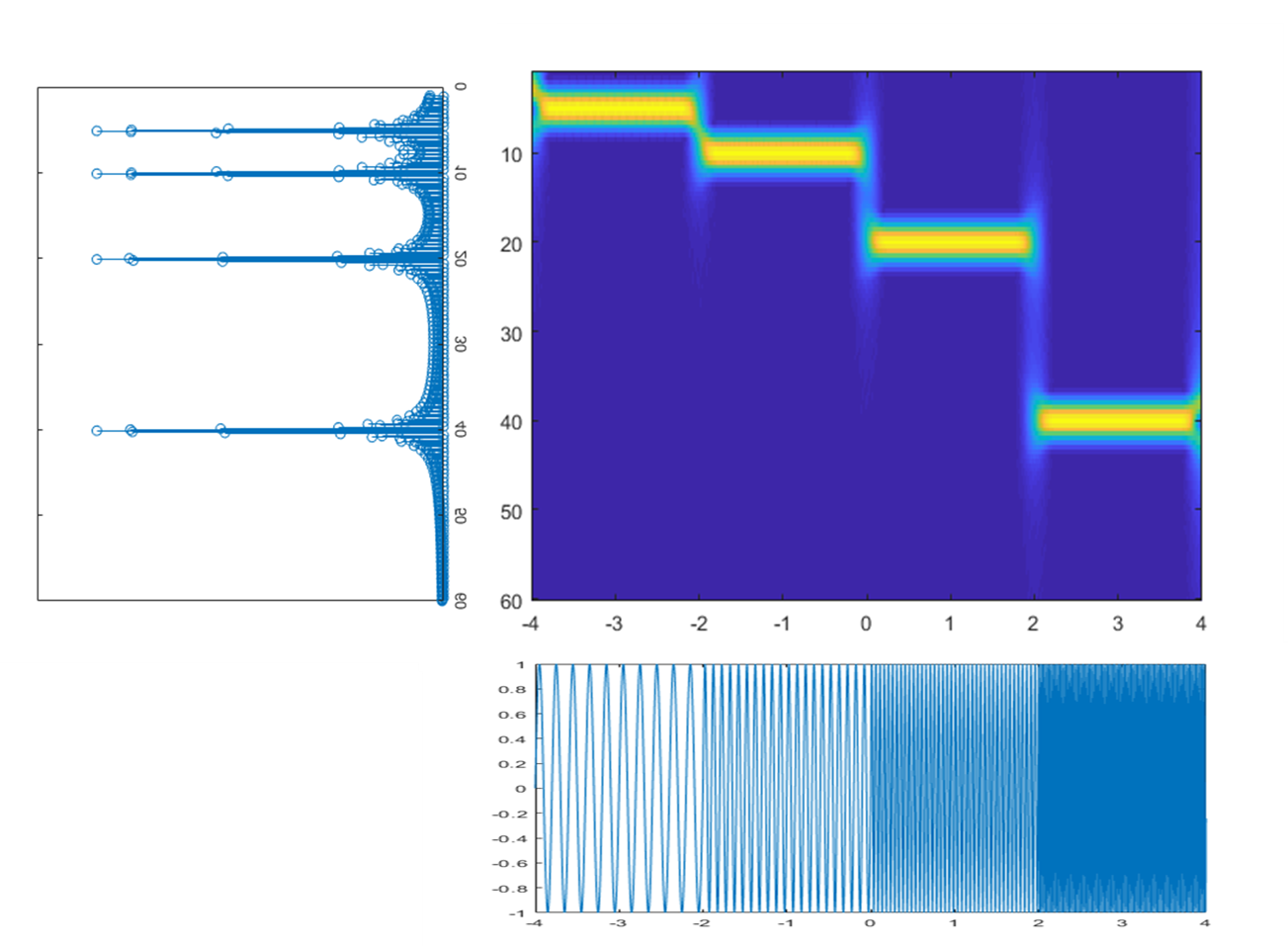1 STFT的基本原理
基本原理可以理解为对一段长信号，截取每一段时间的短信号做fft，将得到的频谱图时间沿时间轴排列，及可得到时频的云图。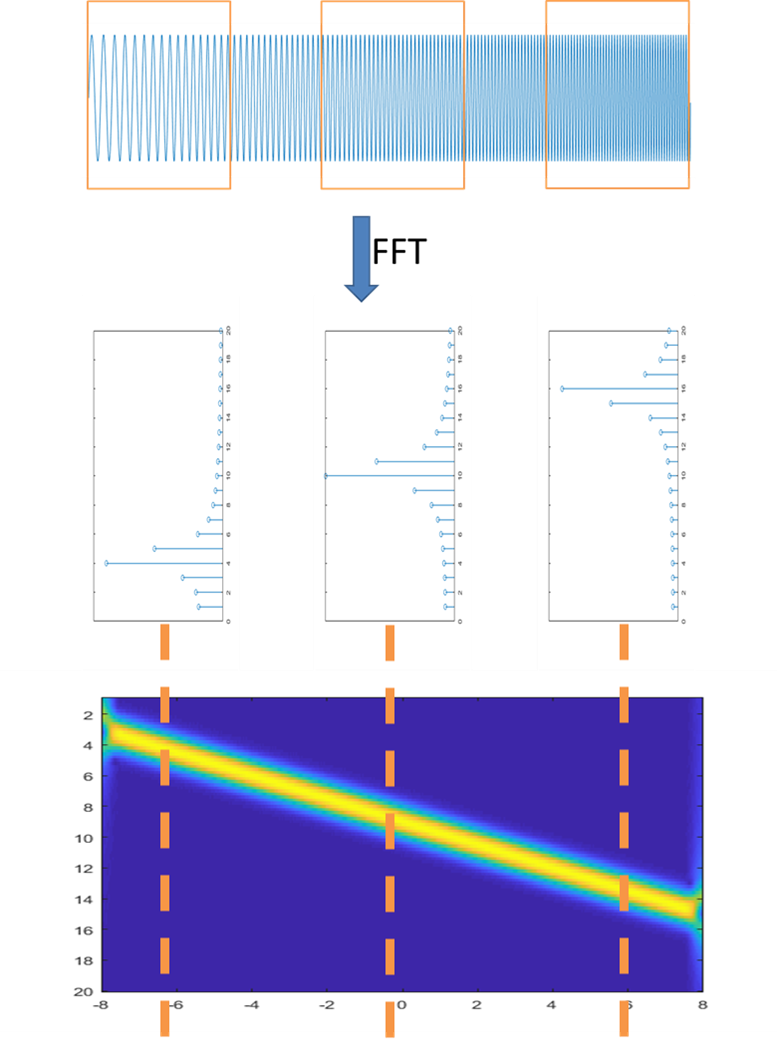2 matlab中实现
这里采用最基础的spectrogram函数作为分析函数。虽然在其它很多地方都能找到能实现更高级用法的函数，比如逆变换： https://ww2.mathworks.cn/matlabcentral/fileexchange/45577-inverse-short-time-fourier-transform-istft-with-matlab 但是这里只是单纯的做可视化分析。
代码如下：
%短时傅里叶变换展示
fs=2^10;    %采样频率 fs=65536hz
dt=1/fs;    %时间精度
timestart=-4;
timeend=4;
t=(0:(timeend-timestart)/dt-1)*dt+timestart;
L=length(t);

%设置信号
z=sin(2*pi*5.*t).*(t<-2)+sin(2*pi*10.*t).*(t>=-2&t<0)+...
sin(2*pi*20.*t).*(t>=0&t<2)+sin(2*pi*40.*t).*(t>=2);
z2=wextend(1,'sym',z,round(length(z)/2));%镜像延拓

wlen=512;%设置窗口长度。窗口越长时间分辨率越差，频率分辨率越好。
hop=1;%每次平移的步长，最小为1。越小图像时间精度越好，但计算量大。
z2=wkeep1(z2,L+1*wlen);%中间截断

%做短时傅里叶
h=hamming(wlen);%设置海明窗的窗长
f=1:0.5:60;%设置频率刻度

[tfr2,f,t2]=spectrogram(z2,h,wlen-hop,f,fs);
tfr2=tfr2*2/wlen*2;
figure
imagesc(t2+timestart-wlen/fs/2,f,abs(tfr2))

3 窗口长度与分辨率
短时傅里叶变换的分辨率和窗口长度wlen的选取，频率的刻度f、平移的步长hop都有关系。
spectrogram函数可以选取较密的频率刻度来增加频域的分辨率，减小平移步长增加时间的分辨率。
但是对于短时傅里叶变换，最重要的还是窗口长度的选取。当频域刻度和平移步长足够密时，增加的只是生成图像的大小，但是物理层面的分辨率却没有改变。改变物理层面分辨率的就是窗口长度。
下图为同一个信号，选取不同窗口长度的图像。其余量不变。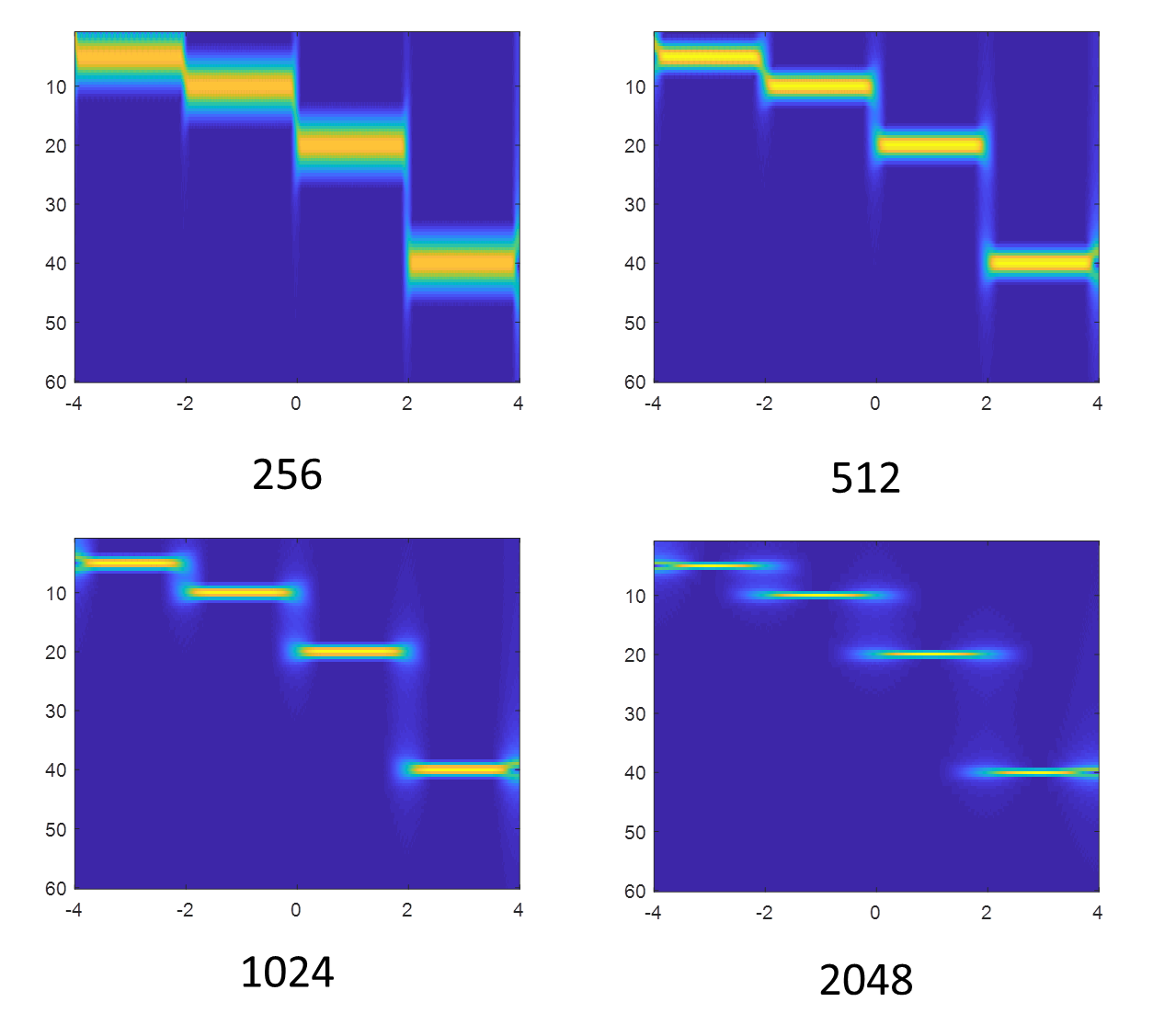窗口长度大的话，比如2048，频率能够清晰的显示出来，但是时间上有些模糊。窗口长度比较小比如256，时间上的分界线能够清晰显示，但是频率的值却不能清晰读出。
这就是窗口的影响。可以想象，当窗口大到整个信号长度时，短时傅里叶变换就退化为傅里叶变换，没有时间维度。当窗口小到单个采样点时，分析也就退化为时域分析，无法做频域分析。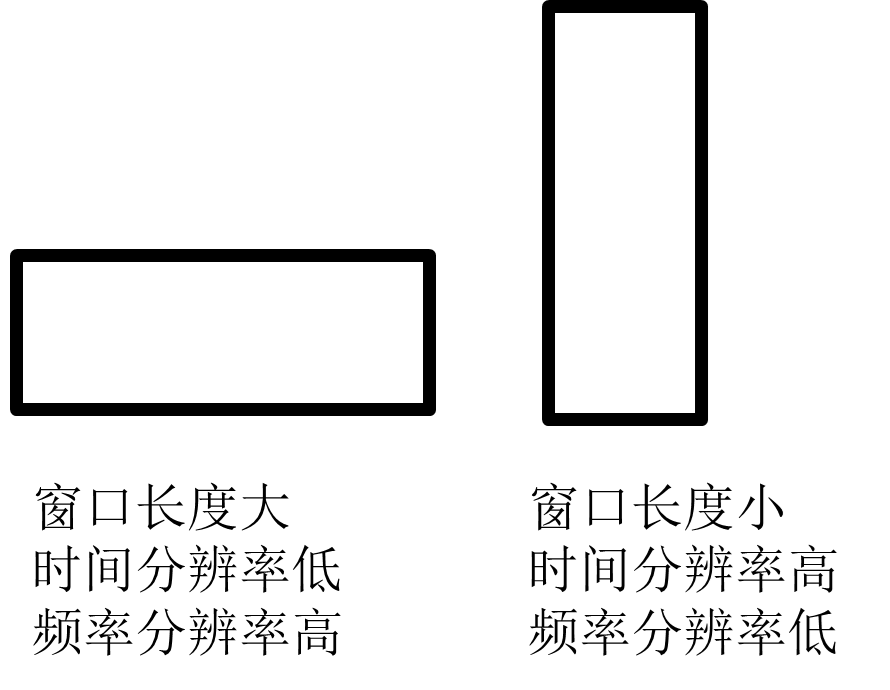4 提高频率分辨率的方法
matlab中的spectrogram函数自带两种提高频率分辨率的方法。
第一种是利用功率谱，将能量集中的原理，提高频率分辨率。 用法为： spectrogram(x,window,noverlap,f,fs,‘reassigned’,‘yaxis’); 前面的同第2节例子，在后面增加了’reassigned’选项。但是这个选项似乎不能用作输出，只能单独以图像形式显示，不知道为什么。
下图为窗函数为256时的两个对比。可以看到右边图的频率分辨率相比左图有了较大提升。但是存在许多噪点。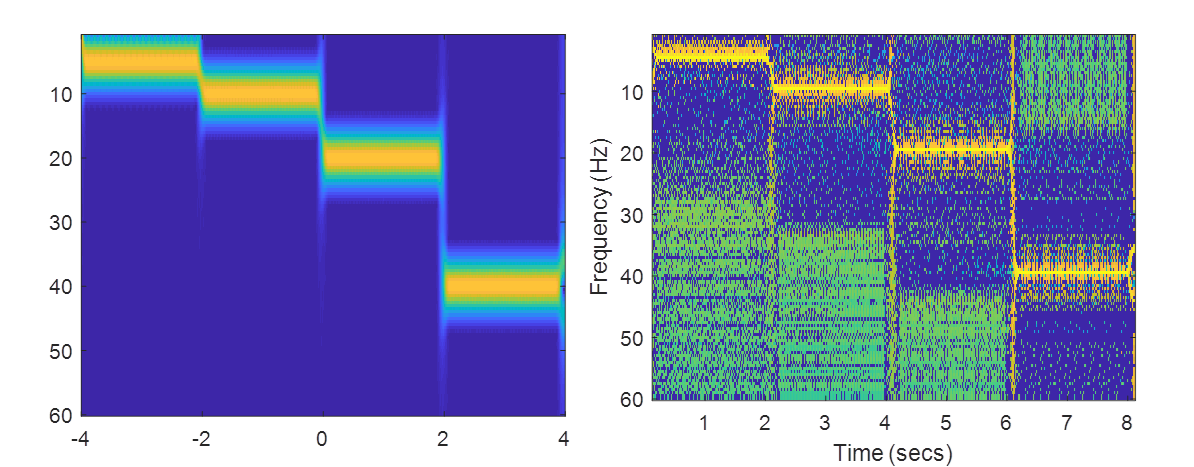第二种是将小于某个数值的数归零，提高频率分辨率。 格式如下： spectrogram(___,‘MinThreshold’,thresh) 参考第2节中的格式如下 spectrogram(z2,h,wlen-hop,f,fs,‘MinThreshold’,-14,‘yaxis’); set(gca,‘YDir’,‘reverse’); 结果如下图所示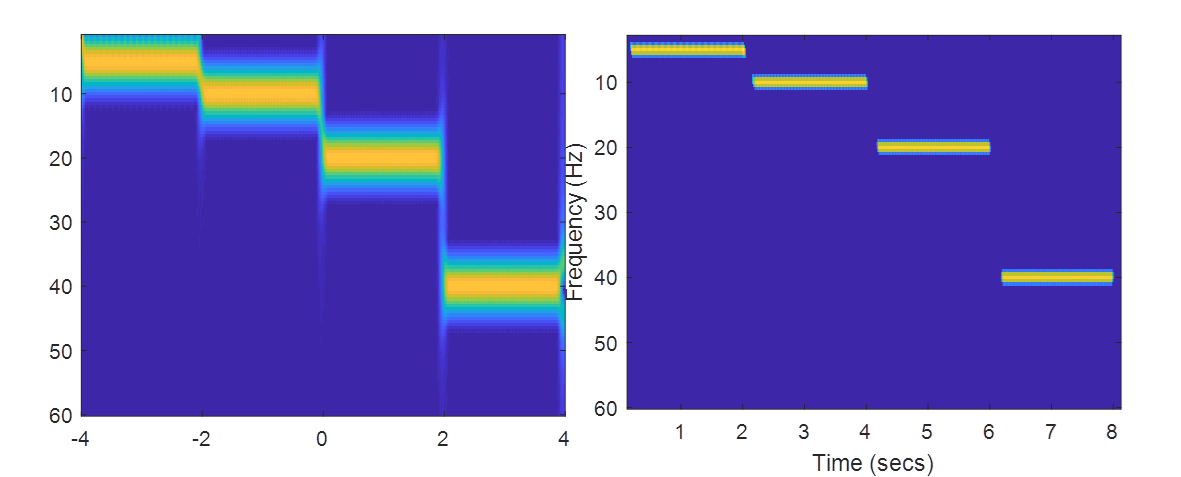5 提取相位信号
短时傅里叶变换不仅可以提取信号的幅值，还可以提取信号的相位。 幅值的提取方法为abs，相位的提取方法为angle。
例：
clear

z=mtlb;
L=length(z);
t=(1:L)/Fs;

z2=wextend(1,'sym',z,round(length(z)/2));
wlen=512;%设置窗长
hop=1;%平移步长
z2=wkeep1(z2,L+1*wlen);%中间截断

h=hamming(wlen);%设置海明窗的窗长
f=100:1:1000;
[tfr2,f,t2]=spectrogram(z2,h,wlen-hop,f,Fs,'reassigned','yaxis');
tfr2=tfr2*2/wlen*2;
figure
imagesc(t,f,angle(tfr2))%提取相位
colormap([linspace(0,1,32)'*ones(1,3);linspace(1,0,32)'*ones(1,3)])

信号的相位图如下：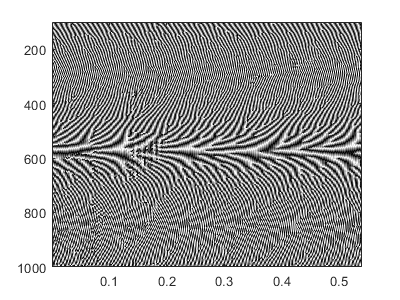展开全文STFT 时频分析 信号处理
• 采用fft、小波分析技术，深入分析地震波频谱特性。便于设计院、振动台试验和科研前期分析环节，如有问题微信公众号咨询：土木科研咨询团
• ## MATLAB 求功率谱

万次阅读 多人点赞 2018-12-13 23:00:42
功率谱 概念 　功率谱（power spectrum）是功率谱密度函数的简称。它定义为单位频带内的信号功率，表示信号功率随着频率的变化情况，即信号功率在频域的分布状况。功率谱表示了信号功率随着频率的变化关系。 　功率...
功率谱
概念
功率谱（power spectrum）是功率谱密度函数的简称。它定义为单位频带内的信号功率，表示信号功率随着频率的变化情况，即信号功率在频域的分布状况。功率谱表示了信号功率随着频率的变化关系。 　　功率谱常用于功率信号（区别于能量信号）的表述于分析，其曲线一般以频率为横坐标，功率为纵坐标。周期性连续星号

x

(

t

)

x(t)

的频谱可以表示为离散的非周期序列

x

n

x_n

，它的幅度频谱的平方

∣

x

n

∣

2

|x_n|^2

所排成的序列，就称之为该信号的功率谱。
定义
功率信号

f

(

t

)

f(t)

在时间段

t

∈

[

−

T

/

2

,

T

/

2

]

t\in[-T/2,T/2]

上的平均功率可以表示为：

(1)

P

=

∫

−

T

/

2

T

/

2

f

(

t

)

2

d

t

P=\int_{-T/2}^{T/2} f(t)^2{\rm d}t \tag{1}

如果

f

(

t

)

f(t)

在时间段

t

∈

[

−

T

/

2

,

T

/

2

]

t\in[-T/2,T/2]

上可以用

f

T

(

t

)

f_T(t)

表示，且

f

T

(

t

)

f_T(t)

的傅里叶变换为

F

T

(

ω

)

=

F

[

f

T

(

t

)

]

F_T(\omega) = F[f_T(t)]

，其中

F

[

]

F[]

表示傅里叶变换。 　　当

T

T

增加时，

F

T

(

ω

)

F_T(\omega)

以及

∣

F

T

(

ω

)

∣

2

|F_T(\omega)|^2

的能量增加。当

T

→

+

∞

T\rightarrow+\infty

时，

f

T

(

t

)

→

f

(

t

)

f_T(t)\rightarrow f(t)

此时

∣

F

T

(

ω

)

∣

2

2

π

T

\frac{|F_T(\omega)|^2}{2\pi T}

可能趋近于一极限，如果该极限存在，其平均功率亦可以在频域表示，即——

(2)

P

=

lim

⁡

T

→

+

∞

1

T

∫

−

T

/

2

T

/

2

f

2

(

t

)

d

t

=

1

2

π

∫

−

∞

+

∞

lim

⁡

T

→

+

∞

∣

F

T

(

ω

)

∣

2

2

π

T

d

ω

P=\lim_{T\rightarrow +\infty}\frac{1}{T} \int_{-T/2}^{T/2}f^2(t){\rm d}t = \frac{1}{2\pi}\int_{-\infty}^{+\infty}\lim_{T\rightarrow +\infty} \frac{|F_T(\omega)|^2}{2\pi T}{\rm d}\omega \tag{2}

定义

∣

F

T

(

ω

)

∣

2

2

π

T

\frac{|F_T(\omega)|^2}{2\pi T}

为

f

(

t

)

f(t)

的功率密度函数，或者简称为功率谱，其表达式如下：

(3)

P

(

ω

)

=

lim

⁡

T

→

+

∞

∣

F

T

(

ω

)

∣

2

2

π

T

P(\omega)=\lim_{T\rightarrow +\infty}\frac{|F_T(\omega)|^2}{2\pi T} \tag{3}

性质
（1） 功率谱密度函数

P

(

ω

)

P(\omega)

是实的； 　　（2）功率谱函数是非负的，即

P

(

ω

)

≥

0

P(\omega)\geq0

； 　　（3）功率谱密度函数的逆傅里叶变换是信号自相关函数

(4)

C

(

τ

)

=

∫

−

∞

+

∞

P

(

ω

)

e

j

ω

τ

d

ω

C(\tau) = \int_{-\infty}^{+\infty}P(\omega)e^{j\omega \tau}{\rm d}\omega \tag{4}

（4）功率谱密度对频率的积分给出信号

f

(

t

)

{f(t)}

的方差，即：

(5)

∫

−

∞

+

∞

P

(

ω

)

d

ω

=

v

a

r

[

f

(

t

)

]

=

E

{

∣

f

(

t

)

−

μ

∣

}

\int_{-\infty}^{+\infty}P(\omega){\rm d}\omega = var[f(t)] = E\{|f(t) - \mu|\} \tag{5}

上式中，

v

a

r

[

]

var[]

表示求方差的算符，

E

{

}

E\{\}

表示求平均的算符，

μ

\mu

表示

f

(

t

)

f(t)

的均值。
MATLAB代码
talk is cheap，show me the code（废话少说，放码过来）
function [pows, freq] = analyse_power_spectrum(X, Fs)
% calculate the FFT of the signal X, transform to power, and
% generate frequency range according to the sampling rate Fs

N = length(X);

% take FFT and shift it for symmetry
amp = fftshift(fft(X));

% make frequency range
fN = N - mod(N, 2);
k = -fN/2 : fN/2 - 1;
T = N / Fs;
freq = k/T;

% select the positive domain FFT and range
one_idx = fN/2 + 2;
amp = amp(one_idx:end);
freq = freq(one_idx:end);

% return power spectrum
pows = abs(amp).^2;
end


其中，输入参数：X是输入的信号，Fs是该信号的采样率，输出参数pows是功率谱，freq是频率谱。下面是一个使用Demo——
fs = 1/6.28;
X = [0:0.01:6.27];
X = sin(X);
[pows,freq] = analyse_power_spectrum(X,30);
figure;
subplot(3,1,1);
plot(X);
title('sin(X)');

subplot(3,1,2);
plot(freq);
title('freuency spectrum');

subplot(3,1,3);
plot(pows,freq);
title('power spectrum');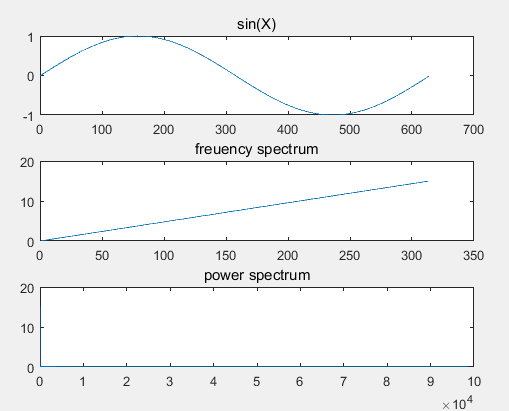展开全文• 以下前半段是我的发现和心得以供参考后半段是我的疑问在此希望有人一起讨论下 一直都是用MATLAB自带pwelch函数来做功率谱最近要把MATLAB转换成C代码然后我就想自己先在MATLAB上用FFT来实现下功率谱为了简单起见welch...
• WaveletAnalysis() 将计算数据集的离散小波变换并提取分割。 对于每个段，计算小波尺度谱和常规功率谱密度。 输出是一个元胞数组，总结了每个段的分析。 提供了示例和文档。
•   在Matlab中，做短时傅里叶变换需要使用函数spectrogram，而在Matlab2019中，引入了一个新的函数stft，下面我们就来看下这两个函数都如何使用。   短时傅里叶变换的基本原理就是将数据分段加窗，做fft，在分段...
  在Matlab中，做短时傅里叶变换需要使用函数spectrogram，而在Matlab2019中，引入了一个新的函数stft，下面我们就来看下这两个函数都如何使用。
短时傅里叶变换的基本原理就是将数据分段加窗，做fft，在分段时会有overlap，因此一个向量的短时傅里叶变换结果是一个矩阵。了解了这点，下面的函数及参数就更加容易理解了。
spectrogram
参数列表
先来看spectrogram函数，在更早期的版本中，这个函数的名字是specgram，几种常用的用法如下：
spectrogram(x)
s = spectrogram(x)
s = spectrogram(x, window)
s = spectrogram(x, window, noverlap)
s = spectrogram(x, window, noverlap, nfft)
s = spectrogram(x, window, noverlap, nfft, fs)
[s, f, t] = spectrogram(x, window, noverlap, nfft, fs)
[s, f, t] = spectrogram(x, window, noverlap, f, fs)
[s, f, t, p] = spectrogram(x, window, noverlap, f, fs)

其中，
x表示输入信号；window表示窗函数，如果window的值是一个整数，那么被分段的x的每一段的长度都等于window，并采用默认的Hamming窗；如果window是一个向量，那么被分段后每一段的长度都等于length(window)，且输入的向量即为所要加的窗函数；overlap表示两段之间的重合点数，overlap的值必须要小于窗长，如果没有指定overlap，默认是窗长的一半，即50%的overlap；nfft表示fft的点数，fft的点数跟窗长可以是不同的，当没有指定该参数时，Matlab会取max(256, 2^(ceil(log2(length(window)))))，即当窗长小于256时，fft的点数是256；当窗长大于256时，fft的点数取大于窗长的最小的2的整数次幂；fs表示采样率，用来归一化显示使用；f表示显示的频谱范围，f是一个向量，长度跟s的行数相同；
当x是实信号且nfft为偶数时，s的行数为(nfft/2+1)当x是实信号且nfft为奇数时，s的行数为(nfft+1)/2当x是复信号时，s的行数为nfft当在输入的参数列表中指定f后，函数会在f指定的频率处计算频谱图，返回的f跟输入的f是相同的； t表示显示的时间范围，是一个向量，长度跟s的列数相同；p表示功率谱密度，对于实信号，p是各段PSD的单边周期估计；对于复信号，当指定F频率向量时，P为双边PSD;如何计算PSD
Examples
首先，生成信号如下，4个点频信号拼接起来：
clc;clear all;close all;
fs = 10e6;
n = 10000;
f1 = 10e3; f2 = 50e3; f3 = 80e3; f4 = 100e3;
t = (0:n-1)'/fs;
sig1 = cos(2*pi*f1*t);
sig2 = cos(2*pi*f2*t);
sig3 = cos(2*pi*f3*t);
sig4 = cos(2*pi*f4*t);

sig = [sig1; sig2; sig3; sig4];

信号的时域波形如下：直接调用spectrogram(sig)，可得如下结果，图中默认横轴是频率，纵轴是时间为了绘图更灵活，我们不直接用spectrogram绘图，而且求出s后，再对s单独绘图，这次我们指定window的大小为256
s = spectrogram(sig, 256);
t = linspace(0, 4*n/fs, size(s,1));
f = linspace(0, fs/2, size(s,2));
figure;
imagesc(t, f, 20*log10((abs(s))));xlabel('Samples'); ylabel('Freqency');
colorbar;noverlap默认是50%，现在我们把它设为window的长度减1，即每次的步进为1
s = spectrogram(sig, 256, 255);
t = linspace(0, 4*n/fs, size(s,1));
f = linspace(0, fs/2, size(s,2));
figure;
imagesc(t, f, 20*log10((abs(s))));xlabel('Samples'); ylabel('Freqency');
colorbar;再加上nfft和fs参数，我们指定fft点数就是窗长
s = spectrogram(sig, 256, 128, 256, fs);

这个的图形跟之前一样，不再画了

如果在返回值中，增加f和t，这样我们下面就不用再重新定义f和t了
[s, f, t] = spectrogram(sig, 256, 128, 256, fs);
figure;
imagesc(t, f, 20*log10((abs(s))));xlabel('Samples'); ylabel('Freqency');
colorbar;

从上面的图中我们可以看出，我们的4个信号的频率都比较小，而画出来的图显示的频谱范围比较大，导致下面很大一部分信息我们其实都不需要。这时，我们就可以通过指定f的区间来计算频谱。为了显示效果更好，我们把其他参数也调一下
window = 2048;
noverlap = window/2;
f_len = window/2 + 1;
f = linspace(0, 150e3, f_len);
[s, f, t] = spectrogram(sig, window, noverlap , f, fs);
figure;
imagesc(t, f, 20*log10((abs(s))));xlabel('Samples'); ylabel('Freqency');
colorbar;最后再把功率谱密度的返回值加上
[s, f, t, p] = spectrogram(sig, window, nfft, f, fs);
figure;
imagesc(t, f, p);xlabel('Samples'); ylabel('Freqency');
colorbar;stft
这个函数在Matlab的解释并不是很多，example也只写了两个，但用法比较简单：
window = 2048;
noverlap = window/2;
nfft = window;
[s, f, t, p] = spectrogram(sig, window, noverlap, nfft, fs);
figure;
imagesc(t, f, 20*log10((abs(s))));xlabel('Samples'); ylabel('Freqency');
title('使用spectrogram画出的短时傅里叶变换图形');
colorbar;

ss = stft(sig,fs,'Window',hamming(window),'OverlapLength',window/2,'FFTLength',nfft);
figure;
imagesc(t, f, 20*log10((abs(ss(1024:end,:)))));xlabel('Samples'); ylabel('Freqency');
title('使用stft画出的短时傅里叶变换图形');
colorbar;欢迎关注微信公众号：Qunat_Times
欢迎大家学习我的课程： System Generator & HLS数字信号处理教程展开全文stft 信号处理
• 编写了BPSK的功率谱密度的MATLAB程序。并得出了运行之后的图形。
• 1、傅里叶变换的幅值称为傅里叶或频谱。 2、F(u)的零值位置与“盒状”函数的宽度W成反比。 3、卷积定理：空间域两个函数的卷积的傅里叶变换等于两个函数的傅里叶变换在频率域中的乘积。f(t)*h(t) <=> H(u)F...
一、先放一些相关的结论：
1、傅里叶变换的幅值称为傅里叶谱或频谱。
2、F(u)的零值位置与“盒状”函数的宽度W成反比。
3、卷积定理：空间域两个函数的卷积的傅里叶变换等于两个函数的傅里叶变换在频率域中的乘积。f(t)*h(t) <=> H(u)F(u)
4、采样定理：如果以超过函数最高频率的两倍的取样率来获得样本，连续的带限函数可以完全地从它的样本集来恢复。
5、严重的混淆甚至会产生完全的误解效果。
6、变化最慢的频率分量(u=v=0)与图像的平均灰度成正比。直流项决定图像的平均灰度。
7、零平均表示存在负灰度，此时图像不是原图像的真实描述，因为所有负灰度为显示目的的都被修剪过。
8、对高通滤波器加一个小常数不会影响尖锐性，但是它的确能防止直流项的消除，并保留色调。
9、在频谱图中,中心部分（uv坐标系中点(0,0)附近）表示原图像中的低频部分。
10、如果原始图像具有十分明显的规律,例如将一个简单图样有规律的平移并填满整个图形,那么其频谱一般表现为坐标原点周围的一圈亮点。
11、将一张灰度图像反相，其频谱的“样式”不变。（个人理解：反相只是将黑白颠倒，但并不改变灰度变化处的对比度）
12、如果频谱图中暗的点数更多，那么实际图像是比较柔和的（因为各点与邻域差异都不大，梯度相对较小）；反之，如果频谱图中亮的点数多，那么实际图像一定是尖锐的、边界分明且边界两边像素差异较大的。
13、高频分量解释信号的突变部分，而低频分量决定信号的整体形象。
所用的傅里叶变换的分析工具是Halcon，代码如下：
read_image (Image, ‘C:/Users/xiahui/Desktop/1.jpg’) fft_image (Image, ImageFFT) 二、不同图像的频谱图分析
左边是原图，右边是经傅里叶变换之后的频谱图。
1、全黑图——频谱图也全黑（图像的分辨率是240*240）2、灰色图——频谱图中央有个单像素的白色的小正方形，坐标是（120,120），值是（30480,0）3、全白图——频谱图中央有个单像素的白色的小正方形，坐标是（120,120），值是（61200,0）4、在图中画一个圆——频谱图呈同心圆状，最中央（坐标120，120）的值为（3852.64,0），其他地方的值有正有负，趋势是越靠近中央值的绝对值越大。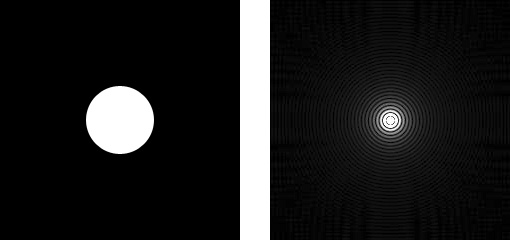5、在图中画一个正方形——最中央的值为（5143.03,0），其他地方的值有正有负，趋势是越靠近中央值的绝对值越大。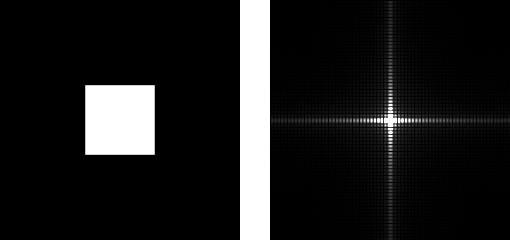6、将上图中正方形旋转45度——最中央的值为（5140.22,0），可认为5140.22≈5143.03；其他地方的值有正有负，趋势是越靠近中央值的绝对值越大。7、画两个圆——最中央的值为（7704.13,0）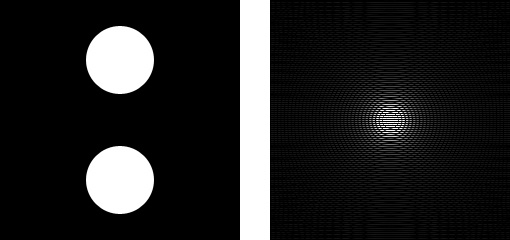8、画一个白圆、一个灰圆——最中央的值为（5772.82,0）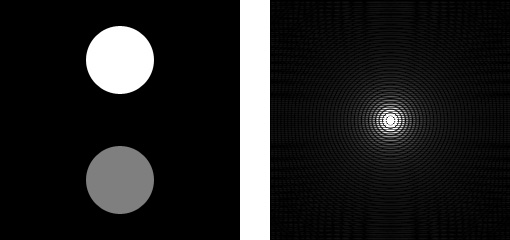9、画四个圆——最中央的值为（15402.8,0）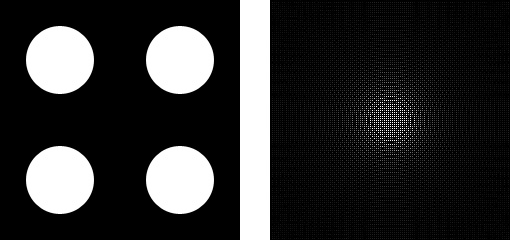10、画2个正方形——最中央的值为（10061.8,0）11、画四个均旋转45度的正方形——最中央的值为（20114.3,0）12、画一条直线——最中央的值为（766,0）13、画一条倾斜15°的线——最中央的值为（876.571,0）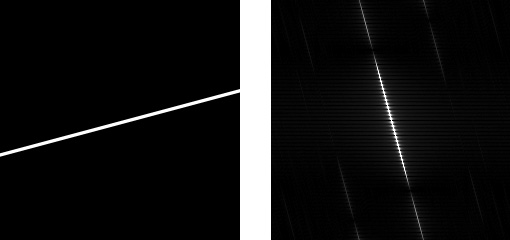14、画一对交叉线——最中央的值为（1194.55,0）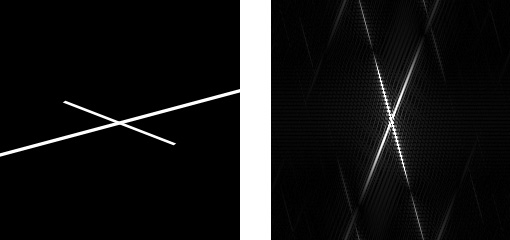15、画一个渐变的圆——虽然也是同心圆，不过没有之前明显了；最中央的值为（1849.6,0）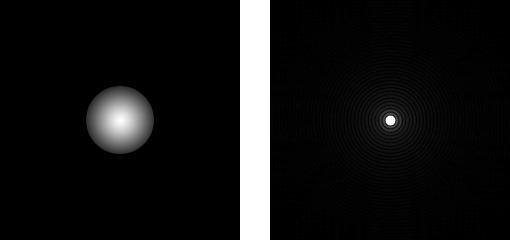16、将整张图用渐变填充——最中央的值为（30470,0），可以认为这个值跟灰色图的值（30480）相等。17、画一个倾斜15°的渐变条——最中央的值为（12051.9,0）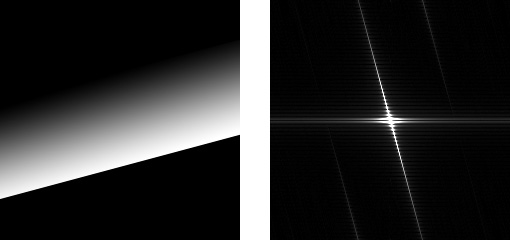18、找来一张图做了不同处理，然后分别观察它们的 频谱图，分别是：
原图、反相图、轻度高斯模糊、重度高斯模糊、平均灰度图、反相平均灰度图。
处理的代码如下：
read_image (Image1, 'C:/Users/xiahui/Desktop/1.jpg')
fft_image (Image1, ImageFFT1)
fft_image (Image2, ImageFFT2)
fft_image (Image3, ImageFFT3)
fft_image (Image4, ImageFFT4)
fft_image (Image5, ImageFFT5)
fft_image (Image6, ImageFFT6)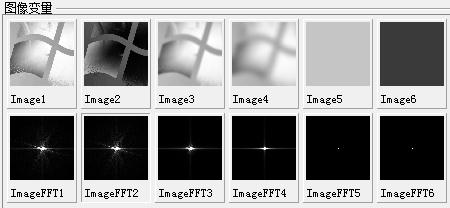由上面可以看出，反相以后，图像的频谱图的“样式”是没有变化的。但其实值是有变化的，这6幅图中央点（120,120）的值分别为：
（47235，0）、（13965，0）、（47169.5，0）、（47130.4，0）、（47280，0）、（13920，0）
根据上面的例子，我们还能得出2个结论：
1、如果图像中有条状的细线，那么沿着此条状细线的走向方向，没有灰度值的变化或变化很小，这样其频谱图就有一条垂直于该条状细线的亮线。这是因为数字图像频谱图的得出和图像的灰度变化（梯度）有关。
2、图像中央点（120,120）的值应该和图像的平均灰度有关，在例3（全白图）中，没有灰度的变化，也就没有频率的变化，所有的能量都集中在频谱图中央的那个单个白色像素块中，其值为61200（图像的分辨率越高，这个极限值越大），在例18中，平均灰度图频谱中央点的值为47280，反相平均灰度图频谱为13920，而47280 + 13920 = 61200。平均灰度图的灰度为198，反相平均灰度图的灰度为57。
出处：http://www.cnblogs.com/xh6300/ 转载于：https://www.cnblogs.com/xh6300/p/5956503.html
展开全文• 利用时域的相对位移等效于频域互功率谱的相位，可以计算出图片的相对偏移量。然后，利用渐进渐出的加权平均法方法消除拼接缝隙，简单且能达到较好的结果。关键词：图像拼接；傅里叶变换；对数极坐标变换1引言图像...算法 计算机视觉 人工智能 机器学习
• ## matlab求单边功率谱

千次阅读 2019-08-13 20:26:25
功率谱有好几种方法，本文例两种，一种是先求信号频谱，然后取平方后平均；第二种是用welch法（可指定各种窗）。 rng default; fs = 1000; % 采样率 t = 0:1/fs:1-1/fs; % x = cos(2*pi*50*t)+ randn(size(t)); x ...
• %% ********************************************** % %% ********************************************** clear;close all;clc; N = 512; xmax = 1; ymax = 1; delta = [0,pi/3]; x = lins相位提取
• 在Windows操作系统下的MATLAB2019b应用程序中编写程序，待程序调试完成后，通过选取两组图像分别进行傅里叶变换处理获取其相应的幅度和相位，并交换两幅图像的幅度和相位重构图像仿真实验来验证图像信号的...图像处理 计算机视觉
• 随机信号及其自相关函数和功率谱密度的MATLAB实现
• 甚至在将 DNA 序列的傅立叶功率谱缩放到序列的最长长度之后，计算不同长度的 DNA 序列的欧几里德距离。 参考Yin, C., & Yau, SST (2015)。 通过傅里叶变换进行全基因组系统发育分析的改进模型。 理论生物学杂志。 ...
• 包含经典功率谱估计：直接法，间接法，Bartlett法，Welch法以及信号的N点傅里叶变换matlab源程序
• 在实际应用中估计信号的频谱（对于随机信号一般是估计其功率谱）是多么重要，我写的这篇文章就是想告诉大家如何在计算机上实现频谱估计。5、需要说明的几点（1）DFT所用的N点离散信号（数据）都是对原有离散信号进行...
• 3)快速傅里叶变换是用Matlab内置函数计算的fft，但对于长度 <1000 点的信号，可以使用嵌套函数 y=Fast_Fourier_Transform(X,N) 。 演示： fs=800； tf=2； t=0:1/Fs:Tf； f=[40 75]; 安培=[4.5 9.22]； 西格玛...
• 目录： 前言 实验环境 Matlab spectrogram函数 1语法 ...3.2鸟声信号功率谱密度追踪 参考： 前言 之前讲了时频分析的原理，现在来讲讲它在matlab里面的实现。 想要复习原理的同学，可以参...
• 讲这个话题，就要先搞清楚频谱、功率谱的概念，可参考我的另一篇文章 信号的频谱 频谱密度 功率谱...做信号处理的朋友应该都会fft比较熟悉，就是求傅里叶变换。我在这里也不再去讲这个函数了，但需要注意的一点 ...fft 能量谱密度
• 只接触很少信号处理的问题，该篇是查阅资料总结的，先对概念等内容进行介绍，最后附matlab功率谱代码。 看了很多资料，没有说明白为啥可以有这么多种方法计算，也不清楚具体这种方法计算出来的是否正确，就写了一...
• 这些方法的MATLAB代码实现参见文章频域特征值提取的MATLAB代码实现（频谱、功率谱、倒频谱）在看这篇文章之前可以参看之前的两篇，其中涉及一些时域特征值介绍和能量、功率信号区别的介绍：Mr.括号：信号的各种时域...
• BB： 最近做脑电信号的时频...Abstract: 我想这篇博文可以帮你弄清楚这几个问题，spectrogram()函数有无返回值调用时图像如何转换，函数输入输出参数的的含义，以及其对图分辨力的影响。 Note: spectrogram()函数...信号处理 stft
• :) FFT频谱分析 短时傅里叶变换 经典功率谱估计（weltch法和Thomson多窗估计法） 现代功率谱估计AR模型法 1.1.jpg 1.1tu.jpg 1.2.jpg...
• 处理信号最常用的三板斧之一的FFT ...% afr.Filename='C:\Users\Hong\Desktop\matlab\城之内.mp3';%读取文件 % afr.SampleRate%文件采样率（只读） % aduioIn.PlayCount=1;%文件读取次数 % afr.O...信号处理 fft 数学建模
• 利用给定的N个样本数据估计一个平稳随机信号的功率谱密度叫做谱估计，功率谱密度一般简称功率谱。谱估计方法分为参数化方法和非参数化方法。非参数化方法又叫经典谱估计，如周期图法、自相关法等，其主要缺点是描述...
• 短时傅里叶变换原理及其MATLAB实现（Short Time Fourier Transform，STFT） 1.短时Fourier变换原理（STFT原理） 信号x(t)短时Fourier变换定义为： 其中w(τ)为窗函数。 X(ω,t)中的时间t表示窗函数w(τ−t)的位置，...
• 信号处理基础——傅里叶变换与短时傅里叶变换 1.FT与STFT概述 2.短时傅里叶变换（Short-time Fourier Transform,STFT） 3.spectrogram函数 4.注意事项 5.实例分析 6.Chirp信号及其生成 7.时频分析实战代码 ...
• 傅里叶变换Fourier transform 1 傅里叶变化基本知识1.1 一维连续Fourier变换对函数f(x)...定义幅值为：定义相位为：用幅值和相位表示傅立叶变换能量谱(或功率谱)现在可以来复习一下傅里叶变换hui gu yi xia:当然了...
• 3.2鸟声信号的功率谱密度 3.2.1平方鸟声信号 3.2.线性鸟声信号 3.3对数鸟声信号 参考： 前言 之前讲了时频分析的原理，现在来讲讲它在matlab里面的实现。 想要复习原理的同学，...时频分析
• ## 功率谱和功率谱密度-MATLAB

千次阅读 多人点赞 2020-05-19 23:15:38
转载于matlab论坛 今天做EEG用到了功率谱...2，pwech方法是计算功率谱密度的，而spectrogram函数，STFT函数，以及tfrstft函数都是计算短时傅里叶变换的，给的是不同时间的频谱值，与功率谱功率谱密度没有关系。 3，在...

# matlab傅里叶变换功率谱matlab 订阅